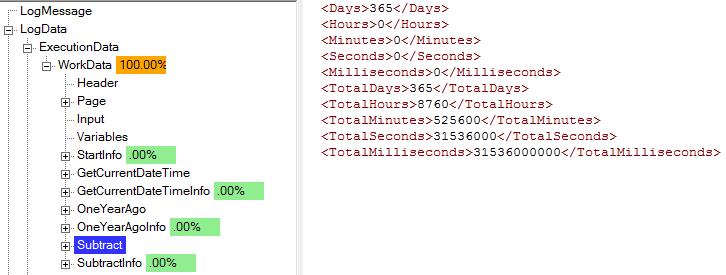Top Top
 Library > EASYProcess Reference Manual 5 > Processes > Services > DateTimeWorkshop > Subtract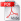Subtract OverviewThe subtract service is helpful to find the amount of time between two dates. This can be a great tool because when dealing with dates, there are certain outliers which can’t easily be accounted for unequal number of days in months, leap years, etc). Thinking of it simply, it performs the following equation: [ToDate]-[FromDate]=[Difference].
 Subtract Use Examples
 Workshop: DateTimeWorkshop Service: Subtract
 Inputs
 FromDate This service performs the following equation: [ToDate]-[FromDate]=[Difference]. This is the FromDate to be used in that equation. This should be the oldest date of the two.
 ToDate This service performs the following equation: [ToDate]-[FromDate]=[Difference]. This is the ToDate to be used in that equation. This should be the most current date of the two.
 Outputs
 Date N/A
 Days If the remainder were returned in A Days, B Hours, C Minutes, D Seconds, E Milliseconds. This is the value A. Together, the Days, Hours, Minutes, Seconds, and Milliseconds makes up the total time between the FromDate and ToDate.
 Hour N/A
 Hours If the remainder were returned in A Days, B Hours, C Minutes, D Seconds, E Milliseconds. This is the value B. Together, the Days, Hours, Minutes, Seconds, and Milliseconds makes up the total time between the FromDate and ToDate.
 LongDate N/A
 Millisecond N/A
 Milliseconds If the remainder were returned in A Days, B Hours, C Minutes, D Seconds, E Milliseconds. This is the value E. Together, the Days, Hours, Minutes, Seconds, and Milliseconds makes up the total time between the FromDate and ToDate.
 Minute N/A
 Minutes If the remainder were returned in A Days, B Hours, C Minutes, D Seconds, E Milliseconds. This is the value C. Together, the Days, Hours, Minutes, Seconds, and Milliseconds makes up the total time between the FromDate and ToDate.
 Second N/A
 Seconds If the remainder were returned in A Days, B Hours, C Minutes, D Seconds, E Milliseconds. This is the value D. Together, the Days, Hours, Minutes, Seconds, and Milliseconds makes up the total time between the FromDate and ToDate.
 ShortDate N/A
 TotalDays The total number of days in the difference between the FromDate and ToDate
 TotalHours The total number of hours in the difference between the FromDate and ToDate
 TotalMilliseconds The total number of milliseconds in the difference between the FromDate and ToDate
 TotalMinutes The total number of minutes in the difference between the FromDate and ToDate
 TotalSeconds The total number of seconds in the difference between the FromDate and ToDateSubtract Use Examples

Basic Use Example

In this example, we are going to look at hardcoded values. During an actual use of this service, it will be with two variables from other process canvas services, but for this case we will look at the year of 2016. This was a leap year, so it is a good example to show how the service handles those date outliers.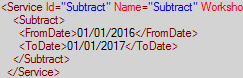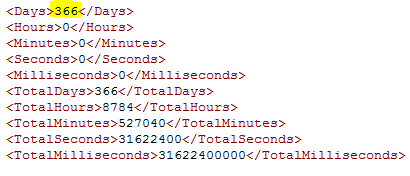The totals also tell us how many minutes, hours, seconds, etc were in that time period.

If we were going to use variables, it could look something like this: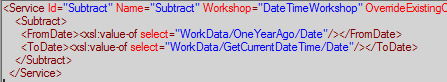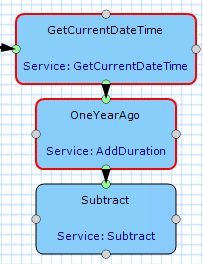The values we are actually using are 12/17/2018 (GetCurrentDateTime) and 12/17/2017 (OneYearAgo). Here is what that output looks like: